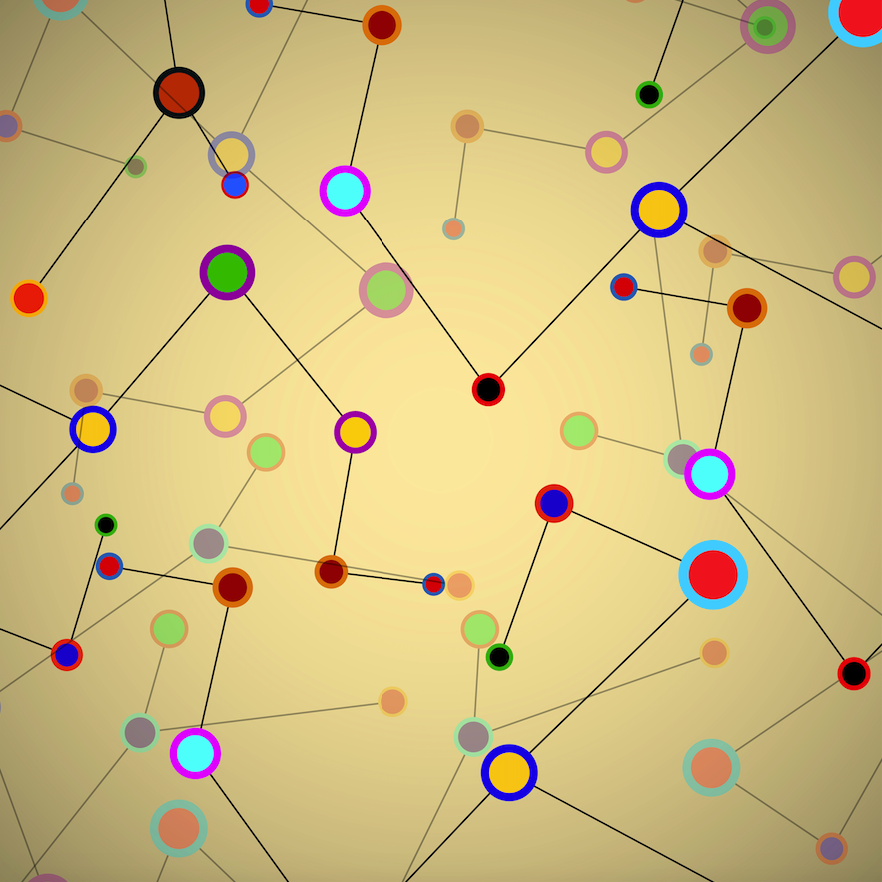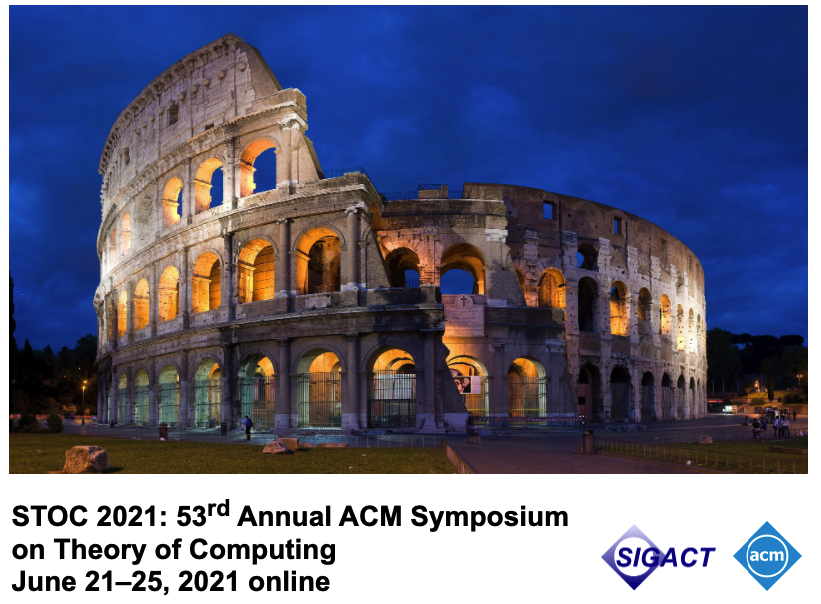# Agenda

A Polynomial-Time Approximation Algorithm for Counting Words Accepted by an NFA
23/junABSTRACT

Counting the number of words of a certain length accepted by a non-deterministic finite automaton (NFA) is a fundamental problem, which has many applications in different areas such as graph databases, knowledge compilation, and information extraction. Along with this, generating such words uniformly at random is also a relevant problem, particularly in scenarios where returning varied outputs is a desirable feature.

The previous problems are formalized as follows. The input of #NFA is an NFA N and a length k given in unary (that is, given as a string 0^k), and then the task is to compute the number of strings of length k accepted by N. The input of GEN-NFA is the same as #NFA, but now the task is to generate uniformly, at random, a string accepted by N of length k.

It is known that #NFA is #P-complete, so an efficient algorithm to compute this function exactly is not expected to exist. However, this does not preclude the existence of an efficient approximation algorithm for it. In this talk, we will show that #NFA admits a fully polynomial-time randomized approximation scheme (FPRAS). Prior to our work, it was open whether #NFA admits an FPRAS; in fact, the best randomized approximation scheme known for #NFA ran in time n^O(log(n)).

Besides, we will mention some consequences and applications of our results. In particular, from well-known results on counting and uniform generation, we obtain that GEN-NFA admits a fully polynomial-time almost uniform generator. Moreover, as #NFA is SpanL-complete under polynomial-time parsimonious reductions, we obtain that every function in the complexity class SpanL admits an FPRAS.

SPEAKERS

Marcelo Arenas, Departamento de Ciencia de la Computación, Universidad Católica de Chile, IMFD.

Luis Alberto Croquevielle, Departamento de Ciencia de la Computación, Universidad Católica de Chile, IMFD.

Rajesh Jayaram, Carnegie Mellon University, USA

Cristian Riveros, Departamento de Ciencia de la Computación, Universidad Católica de Chile, IMFD.

WHEN AND WHERE

Wednesday, June 23, 15.15 hrs (Santiago de Chile)

Fees and registration: https://bit.ly/3zKoB1V